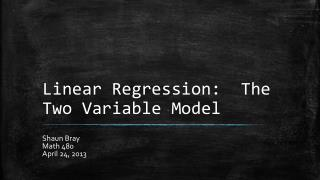DownloadDownload PresentationLinear Regression: The Two Variable Model

# Linear Regression: The Two Variable Model

Télécharger la présentation## Linear Regression: The Two Variable Model

- - - - - - - - - - - - - - - - - - - - - - - - - - - E N D - - - - - - - - - - - - - - - - - - - - - - - - - - -
##### Presentation Transcript

1. Linear Regression: The Two Variable Model Shaun Bray Math 480 April 24, 2013

2. Goal for Today: • What is regression? • Population Regression Function (PRF) • Sample Regression Function (SRF) • The Method of Ordinary Least Squares • How to interpret regression function

3. What is Regression Analysis? • Concerned with the study of the relationship between one variable (dependent) and one or more other variables (independent). • Examples: • Quantity demanded of a commodity in terms of the price of the commodity, income of the consumer, and prices of competing commodities. • Sales of a product related to advertising expenditure incurred.

4. Regression analysis may have one of the following objectives: • To estimate the mean value of the dependent variable, given the values of the independent variables. • To test hypotheses about the nature of dependence. • Predict the mean value of the dependent variable, given the value(s) of the independent variables(s) beyond the sample range. • A combination of one or more of these objectives.

5. Population Regression Function (PRF)

6. Population Regression Function (PRF)

7. Sample Regression Function (SRF) • The PRF is relatively easy to find if we have data of an entire population. • In practice, this is hardly ever the case, so we often look for a sample of a population. • The task here is to estimate the PRF on the basis of the sample information.

8. Sample Regression Function (SRF)

9. Method of Ordinary Least Squares

10. Method of Ordinary Least Squares

11. Conclusion • What is regression analysis and what are its objectives? • Population and Sample Regression Functions • The Method of Ordinary Least Squares